# Rhombuses

Discover the key properties of this unique 4-sided shape.Author
Taylor HartleyExpert Reviewer

Published: August 24, 2023# Rhombuses

Discover the key properties of this unique 4-sided shape.Author
Taylor HartleyExpert Reviewer

Published: August 24, 2023# Rhombuses

Discover the key properties of this unique 4-sided shape.Author
Taylor HartleyExpert Reviewer

Published: August 24, 2023Key takeaways

• A rhombus has four sides – They are 2-D shapes that have all four sides of the same length.
• There are a lot of examples of rhombuses in the real world – Kites are sometimes in a rhombus shape. Road signs and jewelry use rhombuses as shapes as well.
• A rhombus is a parallelogram – A parallelogram is a shape with opposite sides parallel to each other. A rhombus has opposite sides parallel and must also have all four sides equal.

The word rhombus might sound a bit fancy, but it simply describes a four-sided shape, like a diamond, that shares many of the same properties as a square. A diamond-shaped kite is a popular example of a rhombus if it has four equal sides; you may have also seen this shape in certain building designs and floor tiles.

So, what is a rhombus? How can we measure its perimeter or area? Let’s take a look.

## What is a rhombus?The term “rhombus” is derived from the Greek word “rhombos,” which means something that spins.

The definition of a rhombus is a four-sided shape, also known as a quadrilateral, with congruent sides. This means that all sides must be the same length.

All rhombuses are quadrilaterals, but not all quadrilaterals are rhombuses. A quadrilateral is a shape with four sides. For example, a rectangle is a quadrilateral because it has four sides. However, in rectangles, two of the sides are shorter than the others, so while both a rectangle and a rhombus are quadrilaterals, a rectangle is not a rhombus.

Now, you might be thinking “So, are a rhombus and a square the same?”

Squares can be classified as rhombuses because they have four sides of equal length. However, a square must also have four equal angles of 90 degrees, which are known as right angles. A rhombus only needs to have its opposite interior angles equal to each other, as illustrated below.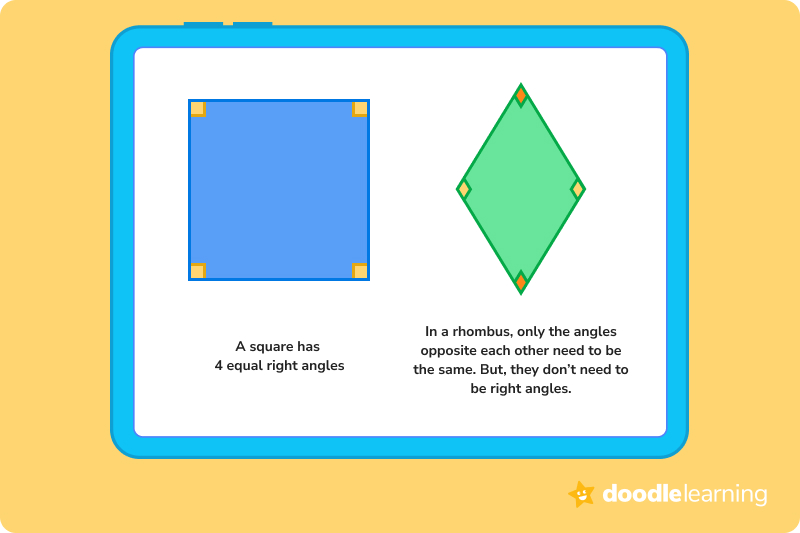So, all squares are rhombuses, but not all rhombuses are squares!

### Real world examples of rhombusesA rhombus is a fairly rare shape in the natural world. However, it can be found in many man-made items. Have you ever played a game of cards? Rhombuses are used to symbolize the suit of diamonds. Some kites are in the rhombus shape too.

Rhombuses are also a popular choice for floor tiles, road signs, Christmas tree decorations, and building designs.## Must-have properties of a rhombusA rhombus is a quadrilateral with unique features that sets it apart from other quadrilaterals, such as a rectangle or a trapezoid.

For a shape to be classified as a rhombus it must:

• Have four sides of equal length
• Have opposite angles be equal. The sum of the adjacent angles will always add up to 180 degrees.
• Have two pairs of parallel sides. This means that if you run a line between opposite vertices, you will always be left with two equal shapes:## Area of a rhombusThe area of a rhombus is described as the amount of space inside the two-dimensional shape. This is usually measured in units squared, for example, in centimeters squared (cm2) or meters squared (m2). Fortunately, because a rhombus has all sides of equal length, finding the area is more straightforward than with other shapes.

We can calculate the area of a rhombus shape in two ways:

1. Multiplying the diagonals of a rhombus and dividing by 2
2. Using base and height measurements

Let’s go through the steps of each of these calculations.

### Using diagonal measurements to find the area of a rhombus1. Multiply the long diagonal measurement (imagine a line going right through the middle of a rhombus lengthways), and the short diagonal measurement of the shape: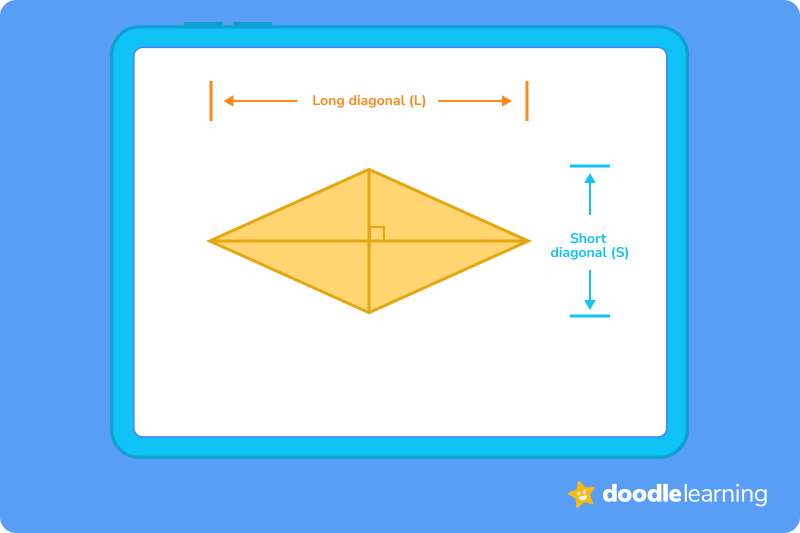1. So, let’s say that the long diagonal measurement is 8 cm, and the short diagonal measurement is 6 cm. To find the area of our rhombus, we first need to multiply both measurements together:

8 x 6 = 48

1. Now, this is the same way that we would work out the area of a rectangle: height x length. This is illustrated in the diagram below by drawing an imaginary rectangle around the rhombus: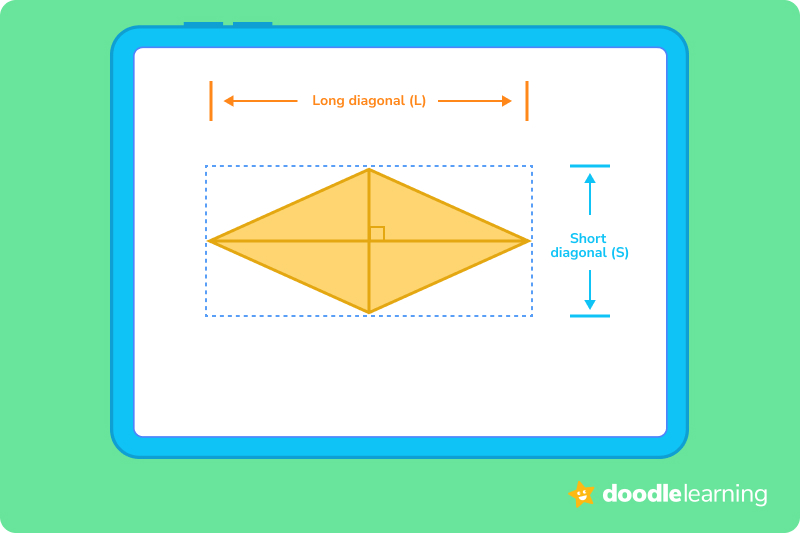1. This diagram shows that the area of the rhombus is smaller than the area of the rectangle. So, to work out the area of the rhombus, we then need to divide the answer by 2 because a rhombus is half the area of our imaginary rectangle. We can see this when we fold the rhombus in half, as shown below: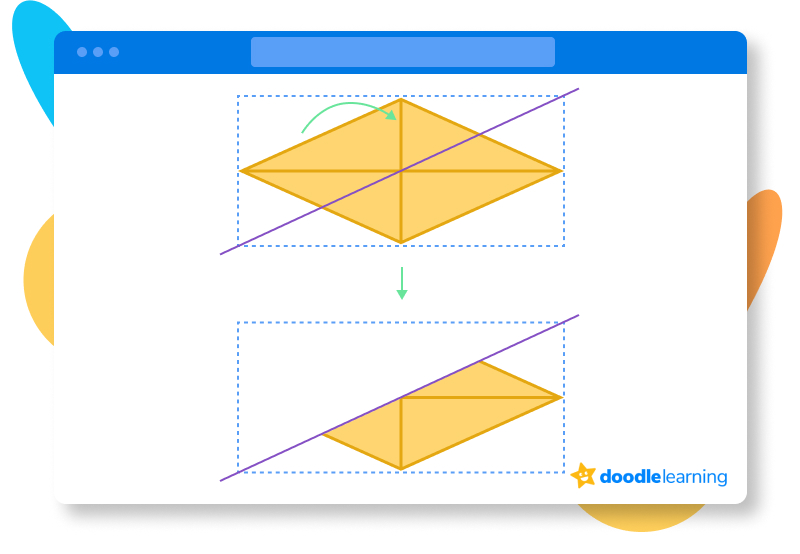So, we divide the original answer by 2:

48 ÷ 2 = 24

The area of the rhombus is 24 cm2.

### Using base and height measurements to find the area of a rhombus1. For this method, we need to measure the base (the length of one of the sides) and the height of a rhombus: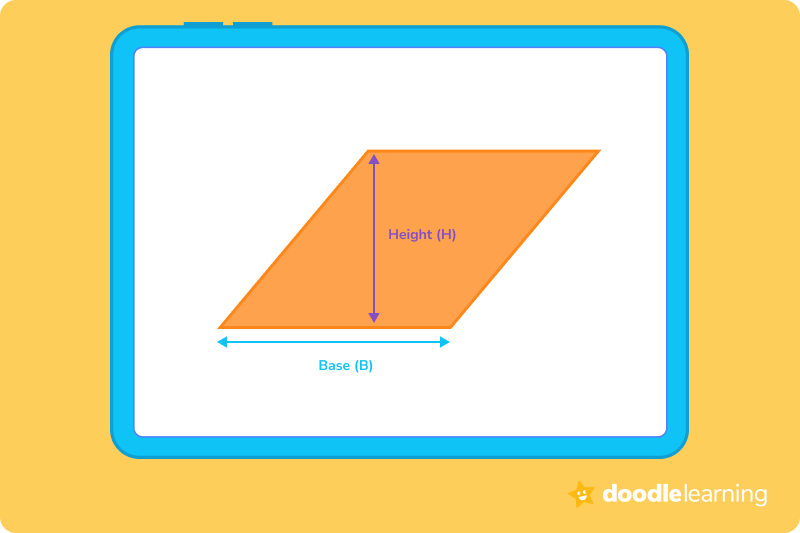1. In this example, the base measurement is 5 cm, and the height measurement is 7 cm. Just as in the previous example, we need to multiply both measurements together:

5 x 7 = 35

1. When we calculate the base and the height of a rhombus shape, we don’t need to divide the answer by 2 because we are calculating the exact shape of the rhombus rather than an imaginary rectangle around it. So, in this example, the area of the rhombus is 35 cm2.

## Perimeter of a rhombusThe perimeter of a rhombus is the total length of its boundaries. Basically, it’s the sum of all the side lengths. We know that all four sides of a rhombus are of equal length, which makes this a fairly straightforward calculation.

1. First, we need to measure the length of one side: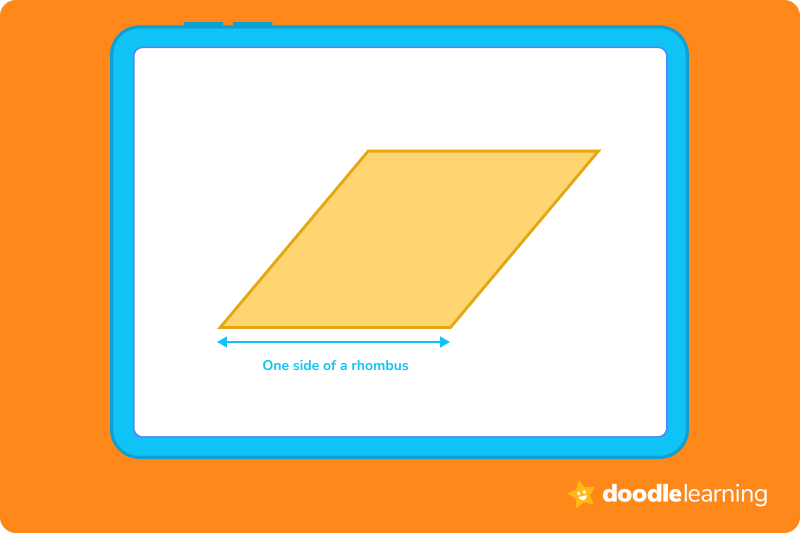1. Remember, we can pick any side to measure because all sides of a rhombus are the same length. In this example, let’s say the length of one of the sides is 4 cm.
2. Now, we just need to multiply the answer by 4, because there are 4 sides to a rhombus:

4 x 4 = 16

1. So, the perimeter of our rhombus is 16 cm.

## Let’s practice together!### 1. Identify the rhombus.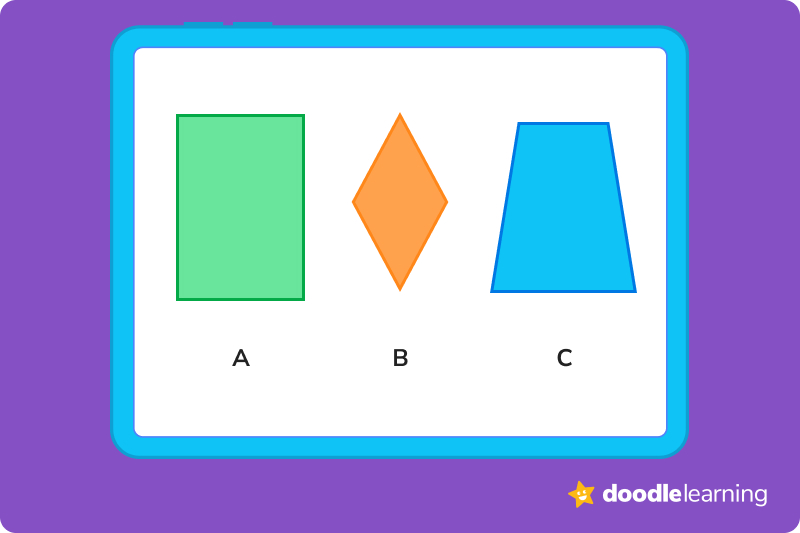The correct answer is B because this is the only shape with 4 sides of equal length.

### 2. Calculate the area of the rhombus.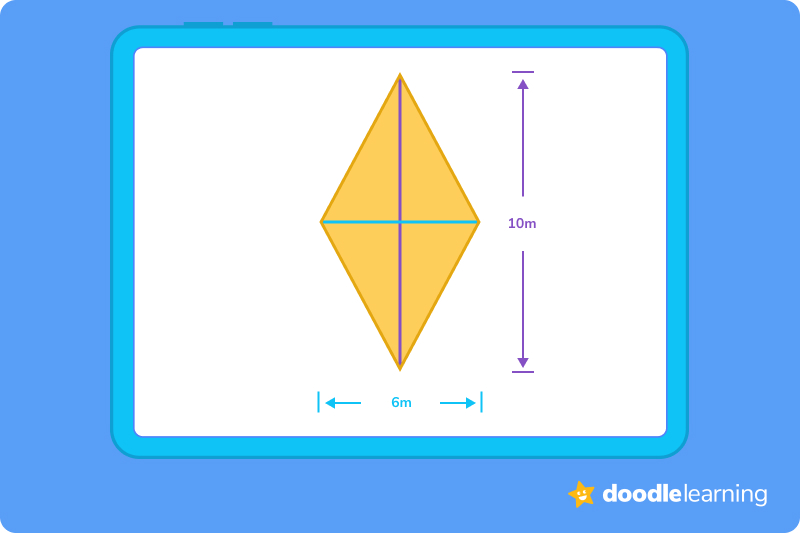In this problem, we know the long and the short diagonal measurements of the rhombus. So, first, we need to multiply these two numbers together:

6 x 10 = 60

Now, because we are using diagonal measurements, we need to divide the answer by 2:

60 ÷ 2 = 30

Therefore, our answer is 30 m2.

### 3. What is the perimeter of the rhombus?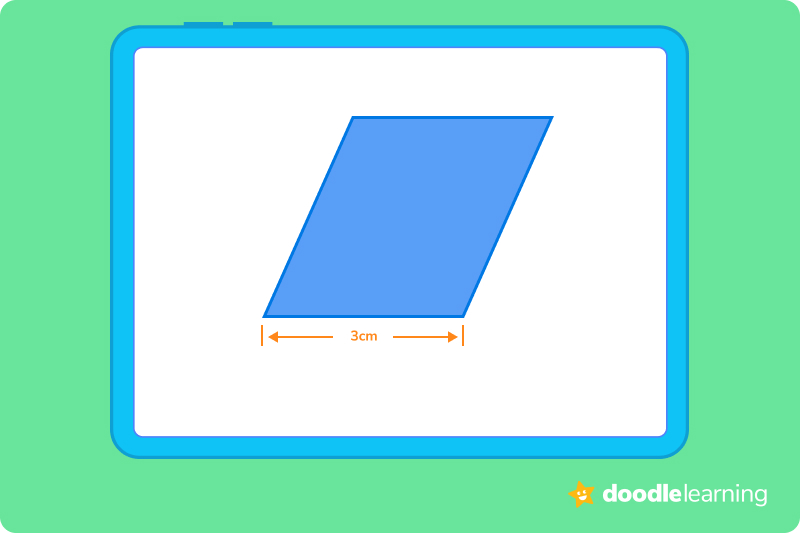All four sides of a rhombus are the same length. So, if we know that one side is 3 cm, we just need to multiply this number by 4:

4 x 3 = 12

So, the correct answer is 12 cm.

Ready to give it a go?

Now it’s time to put your knowledge to the test! Work through the following problems on your own, and see how you do. Don’t feel disheartened if you struggle with any of the questions. Remember, practice makes perfect!

Feel free to look back through this guide to refresh your memory whenever you need to.

## Practice problems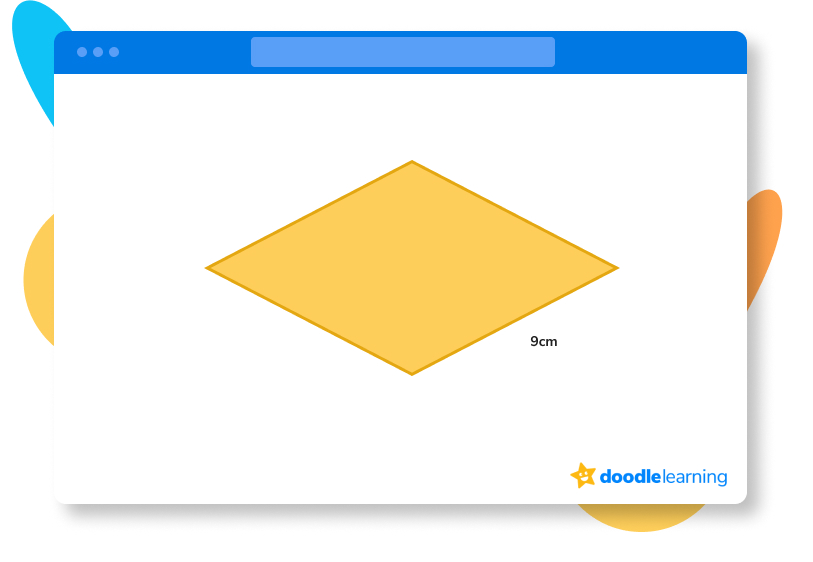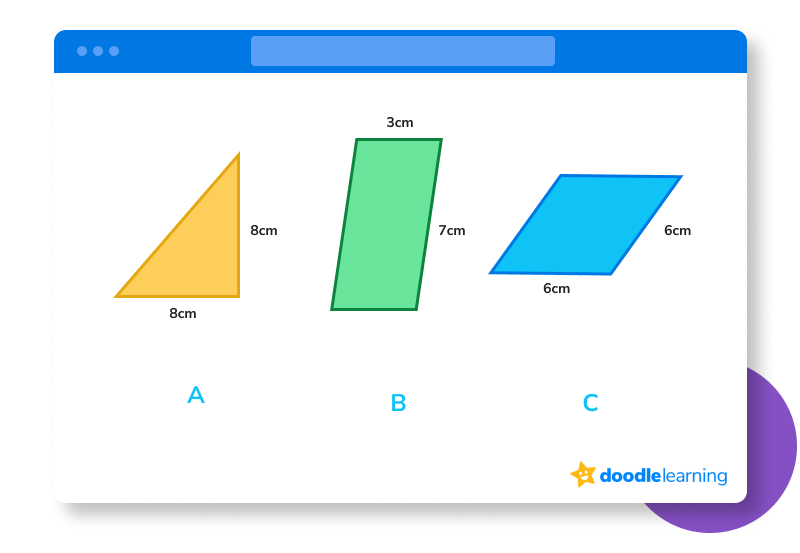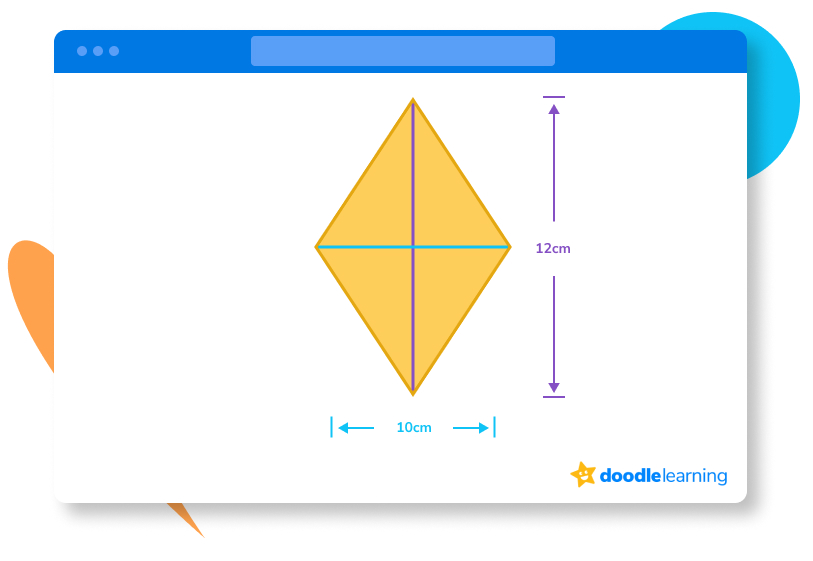## Parent Guide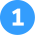How did we get here?

We have the base measurement and the height measurement of the rhombus in this example. So, all we need to do is multiply base times height:

8 x 6 = 48

This makes the answer 48 cm2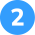How did we get here?

A rhombus has 4 sides of equal length. So, if we know that one side is 9 cm, we just need to multiply this number by 4:

9 x 4 = 36

Therefore, the answer is 36 cm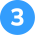How did we get here?

In a rhombus, only the opposite angles must be the same. If a shape has four equal sides and 4 equal angles, then it’s a square.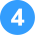How did we get here?

1. A rhombus must have 4 sides of equal length. Shape A only has 3 sides and shape B has 4 sides but only its opposite sides are of equal length.
2. Shape C is the rhombus because it has 4 sides of equal length.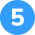How did we get here?

1. To find the area of the rhombus in this example, we first need to multiply the long diagonal measurement (12 cm) with the short diagonal measurement (10 cm):

12 x 10 = 120

1. Now, the area of a rhombus is half the product of its diagonal measurements. So, we just need to divide our answer by 2:

120 ÷ 2 = 60

1. This gives us our answer of 60 cm2

## FAQs about math strategies for kidsWe understand that diving into new information can sometimes be overwhelming, and questions often arise. That’s why we’ve meticulously crafted these FAQs, based on real questions from students and parents. We’ve got you covered!

Yes, a rhombus must have four sides of equal length. A rhombus must also have its opposite sides parallel and opposite angles equal.

For a shape to be a rhombus, it has to have four straight sides of equal length and the opposite angles need to be the same. The most common rhombus shape is a diamond. However, technically, a square can also be classified as a rhombus because it has four sides of equal length and the opposite angles are equal. However, it is important to note that not all rhombuses are squares because rhombuses don’t all have 90° angles.

If a rhombus is a square then it must have 4 right angles. However, for a shape to be classified as a rhombus, it only needs to have the opposite angles equal, and it is not required that they are right angles.

There are certain properties that a shape must have to be a rhombus:

• Opposite angles must be equal
• All four sides of the shape need to be the same length
• The opposite sides of a rhombus must be parallel to each other## Related Posts

Title 1

Title 2

Title 3

Lesson credits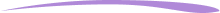Taylor Hartley

Taylor Hartley is an author and an English teacher based in Charlotte, North Carolina. When she's not writing, you can find her on the rowing machine or lost in a good novel.

Jill Padfield has 7 years of experience teaching high school mathematics, ranging from Alegra 1 to AP Calculas. She is currently working as a Business Analyst, working to improve services for Veterans while earning a masters degree in business administration.

Taylor Hartley

Taylor Hartley is an author and an English teacher based in Charlotte, North Carolina. When she's not writing, you can find her on the rowing machine or lost in a good novel.

Jill Padfield has 7 years of experience teaching high school mathematics, ranging from Alegra 1 to AP Calculas. She is currently working as a Business Analyst, working to improve services for Veterans while earning a masters degree in business administration.# Are you a parent, teacher or student?

Are you a parent or teacher?

## Hi there!

Book a chat with our team